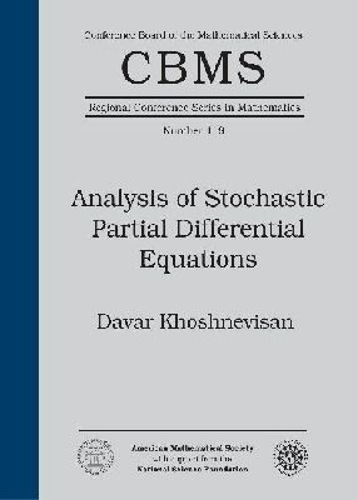Find your perfect holiday reading•# Analysis of Stochastic Partial Differential Equations - CBMS Regional Conference Series in Mathematics (Paperback)

(author)
£35.50
Paperback 116 Pages / Published: 30/06/2014
• We can order this

Usually dispatched within 2 weeks

• This item has been added to your basket
The general area of stochastic PDEs is interesting to mathematicians because it contains an enormous number of challenging open problems. There is also a great deal of interest in this topic because it has deep applications in disciplines that range from applied mathematics, statistical mechanics, and theoretical physics, to theoretical neuroscience, theory of complex chemical reactions [including polymer science], fluid dynamics, and mathematical finance.

The stochastic PDEs that are studied in this book are similar to the familiar PDE for heat in a thin rod, but with the additional restriction that the external forcing density is a two-parameter stochastic process, or what is more commonly the case, the forcing is a ``random noise,'' also known as a ``generalized random field.'' At several points in the lectures, there are examples that highlight the phenomenon that stochastic PDEs are not a subset of PDEs. In fact, the introduction of noise in some partial differential equations can bring about not a small perturbation, but truly fundamental changes to the system that the underlying PDE is attempting to describe.

The topics covered include a brief introduction to the stochastic heat equation, structure theory for the linear stochastic heat equation, and an in-depth look at intermittency properties of the solution to semilinear stochastic heat equations. Specific topics include stochastic integrals a la Norbert Wiener, an infinite-dimensional Ito-type stochastic integral, an example of a parabolic Anderson model, and intermittency fronts.

There are many possible approaches to stochastic PDEs. The selection of topics and techniques presented here are informed by the guiding example of the stochastic heat equation.

Publisher: American Mathematical Society
ISBN: 9781470415471
Number of pages: 116
Dimensions: 254 x 178 mm

## Reviews

Sign In To Write A Review

Please sign in to write a review

Your review has been submitted successfully.